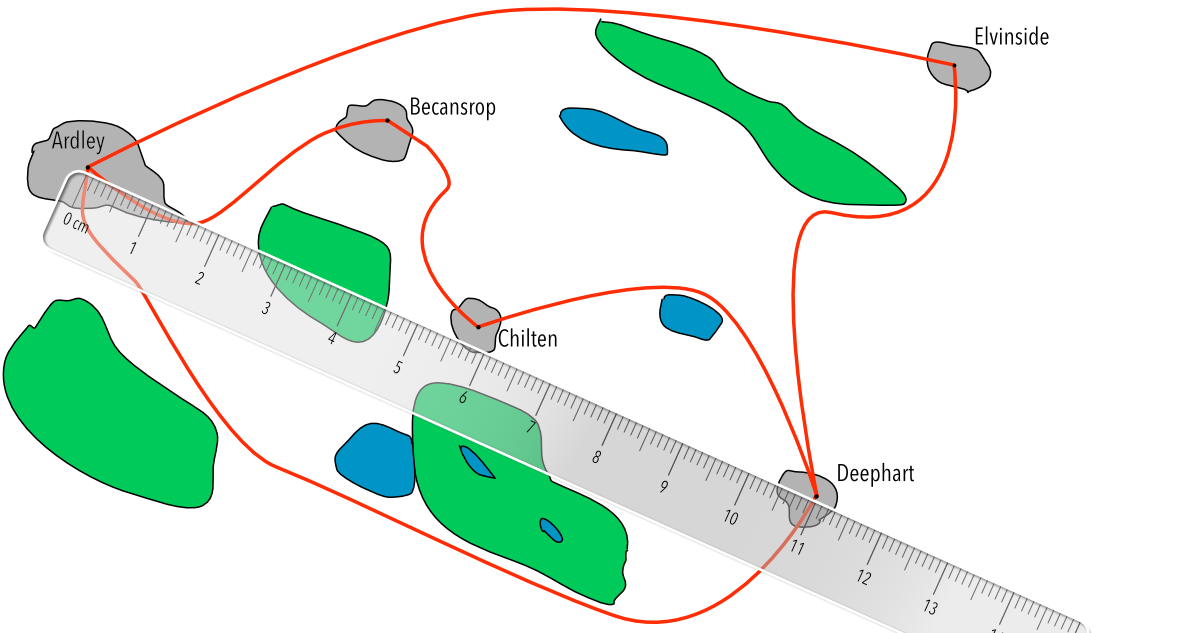Scales in Maps

# Scales in Maps

GCSE(F),

Maps are drawn to a scale. Map scales are either given as a ratio, such as 1:2500, or given as a measured distance on the map representing an actual distance, such as 1 centimetre represents 5 kilometres.

## Examples

1. What, in real life, is the distance from Ardley to Deephart? On the map, 2cm represents 5km.The ruler measures 11cm. This is a multiple of 5.5 (from 2cm to 11cm). Multiply 5km by 5.5 = 27.5km

MapActual
Scale:2 cm5km
x 5.5
Measured:11cm

2. What is the straight-line distance from Ardley to Becansrop, if the distance is 10.75km in real life?